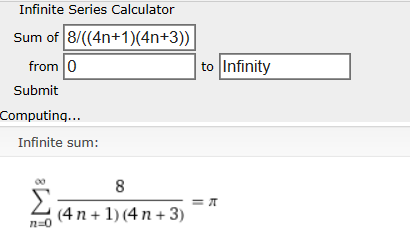# Infinite Series that $= \pi$ or $= \pi^2$

Yesterday night, I was looking for an infinite series that equals to $\pi$. And I found one! Using WolframAlpha's Infinite Series Calculator, I got:It was derived from: $\displaystyle\sum_{n=0}^{\infty} \frac{1}{(4n + 1)(4n + 3)} = \frac{\pi}{8}$

I also found an infinite series that converges to $\pi^2$:

$\displaystyle\sum_{n=0}^{\infty} \frac{6}{(n + 1)^2} = \pi^2$

It was derived from:

$\displaystyle\sum_{n=0}^{\infty} \frac{1}{(n + 1)^2} = \frac{\pi^2}{6}$

I hope you enjoyed this article and comment if you find any other series that converges to $\pi^2$ or $\pi$ - must be in LaTeX!

# AlgebraNote by A Former Brilliant Member
1 year ago

This discussion board is a place to discuss our Daily Challenges and the math and science related to those challenges. Explanations are more than just a solution — they should explain the steps and thinking strategies that you used to obtain the solution. Comments should further the discussion of math and science.

When posting on Brilliant:

• Use the emojis to react to an explanation, whether you're congratulating a job well done , or just really confused .
• Ask specific questions about the challenge or the steps in somebody's explanation. Well-posed questions can add a lot to the discussion, but posting "I don't understand!" doesn't help anyone.
• Try to contribute something new to the discussion, whether it is an extension, generalization or other idea related to the challenge.
• Stay on topic — we're all here to learn more about math and science, not to hear about your favorite get-rich-quick scheme or current world events.

MarkdownAppears as
*italics* or _italics_ italics
**bold** or __bold__ bold
- bulleted- list
• bulleted
• list
1. numbered2. list
1. numbered
2. list
Note: you must add a full line of space before and after lists for them to show up correctly
paragraph 1paragraph 2

paragraph 1

paragraph 2

[example link](https://brilliant.org)example link
> This is a quote
This is a quote
    # I indented these lines
# 4 spaces, and now they show
# up as a code block.

print "hello world"
# I indented these lines
# 4 spaces, and now they show
# up as a code block.

print "hello world"
MathAppears as
Remember to wrap math in $$ ... $$ or $ ... $ to ensure proper formatting.
2 \times 3 $2 \times 3$
2^{34} $2^{34}$
a_{i-1} $a_{i-1}$
\frac{2}{3} $\frac{2}{3}$
\sqrt{2} $\sqrt{2}$
\sum_{i=1}^3 $\sum_{i=1}^3$
\sin \theta $\sin \theta$
\boxed{123} $\boxed{123}$

## Comments

Sort by:

Top Newest

$\pi$=$\sum_{n=1}^{\infty}\frac{\Gamma(n-1/2)\Gamma(1/2+1)}{\Gamma(n+1)}$.Hope you like it.

- 1 year ago

Log in to reply

Log in to reply

The proof relies on the iterated properties of beta function.Check the discussion.

- 1 year ago

Log in to reply

I did - it's great but I am in Year 10, becoming Year 11. How can I do calculus then?

Log in to reply

Well I didn't discovered calculus until I was at last year of becoming 10.You know it's never too late to learn something new.

- 1 year ago

Log in to reply

×

Problem Loading...

Note Loading...

Set Loading...